# Preparation of Nano Pore ZSM-5 Membranes: Experimental, Modeling and Simulation

Document Type : Original Research Paper

Authors

1 Department of Chemical Engineering, Malek-Ashtar University of Technology, Tehran, Iran

2 Department of Chemical Engineering, South Tehran Branch, Islamic Azad University, Tehran, Iran

Abstract

Nano pore ZSM-5type membranes were prepared on the outer surface of a porous-mullite tube by in situ liquid phase hydrothermal synthesis. The hydrothermal crystallization was carried out under an autogenous pressure, at a static condition and at a temperature of 180°C with tetra propyl ammonium bromide (TPABr) as a template agent. The molar composition of the starting gel of ZSM-5 zeolite membrane was: SiO2/Al2O3=100, Na2O/Al2O3=0.292, H2O/Al2O3=40–65, TPABr/ SiO2=0.02-0.05. The zeolites calcinations were carried out in the air at 530°C, to burn off the template (TPABr) within the zeolites. X-ray diffraction (XRD) patterns of the membranes consisted of peaks corresponding to the support and zeolite. The crystal species were characterized by XRD, and morphology of the supports subjected to crystallization was characterized by scanning electron microscopy (SEM). Performance of Nano-porous ZSM-5 membranes was studied for separation of water–unsymmetrical dimethylhydrazine (UDMH) mixtures using pervaporation (PV). Finally, a comprehensive unsteady-state model was developed for the pervaporation of water-UDMH mixture by COMSOL Multiphysics software version 5.2. The developed model was strongly capable of predicting the effect of various dimensional factors on concentration and velocity distributions within the membrane module. The best ZSM-5 zeolite membranes had a water flux of 2.22 kg/m2.h at 27°C. The best PV selectivity for ZSM-5 membranes was obtained to be 55.

Keywords

#### Full Text

INTRODUCTION

Dehydration of organic solvents is presently the major market of PV. High separation factors and water permeate fluxes are reported in previous studies on pervaporation dehydration of isopropanol, ethanol, n-butanol, n-butyl-acetate, ethylene glycol and acetic acid aqueous solution [1-4]. Uragami et al. (2015) investigated the effect of immersion time in CaCl2 or MgCl2 methanol solutions on the permeation flux and separation factor of pervaporation dehydration of ethanol aqueous solution using Alg-DNA/Mg2+ membrane. Their results showed that after immersing the membrane in methanol solution for 12 hours, the separation factor increased significantly, while decreased after 12 hours [1, 2, 5-8].

Zeolite membranes are usually used in pervaporation processes due to their strong potential. These membranes are synthesized using various techniques such as hydrothermal in-situ crystallization, chemical vapor phase technique and spray seed coating. Zeolite NaA membrane was reported to be excellent materials for solvent dehydration by PV. But under slightly severe conditions and under hydrothermal stresses, zeolite NaA membranes behaved unsuitably due to hydrolysis. There are only a few attempts to develop hydrophilic highly siliceous zeolite membranes of different Si/Al ratios with improved hydrothermal stabilities.

Many studies have been done to model concentration distribution within the membrane module in order to commercialize PV separation systems [3, 4, 9, 10]. There are two major approaches to PV simulations: Molecular Dynamic (MD) simulation and Computational Fluid Dynamic (CFD) simulation. Based on MD, Huang et al. (2014) developed a model to explain free-volume form and the flexibility and stiffness of polymer chain. Their results obtained from MD simulations were in good agreement with the chemical structure of the polyelectrolyte complex membranes (PECMs). Jain et al. (2017) developed a mathematical model for tubular pervaporation membrane module for separation of n-heptane/thiophene model gasoline. Their results showed that the dimensional factors had positive effects on separation performance of pervaporation membranes [11-15].

Based on CFD simulation, Moulik et al. (2016) developed a steady state model to predict concentration distribution within the membrane module in pervaporation of acetic acid solution . Their results were in good agreement with experimental data, but their model was not comprehensive since time-dependency of the concentration distribution within the membrane module was neglected. They also didn’t model the concentration distribution within feed section, which significantly affects the concentration profile in membrane side. Prasad et al. (2016) also developed a 2D steady-state model using CFD technique. They also modeled only membrane section and assumed the conditions to be a steady state .

As understood, a comprehensive transient model is required, is capable of predicting concentration distribution within both membranes and feed sections. In this paper, preparation methods of the Nano pore ZSM-5 zeolite membrane on mullite support are reported. Performances of the membranes prepared by hydrothermal in situ crystallization were studied in the separation of the water–UDMH by PV. Finally, a complete transient 2D model was developed based on solving Navier-Stokes equations of mass and momentum transfer, simultaneously. The conservation equations were solved using COMSOL Multiphysics software version 5.2. COMSOL applies finite element method (FEM) to solve the equations numerically. Effect of various membrane dimensions and separation times was investigated to find the optimum operating conditions. The transient model obtained here was distinctively capable of predicting concentration distribution of water through both membranes and feed sides of the separation module. The results indicated that the time-dependent study is necessary and neglecting this dependency is a serious mistake in pervaporation simulations. The results also confirmed that the effect of dimensional factors related to membrane module geometry on concentration distribution is very important and cannot be neglected.

EXPERIMENTAL

Materials

In this study, mullite supports were thermally created from kaolin clay using the high-temperature calcination method. Kaolin (SL-KAD grade) has been supplied by WBB cooperation, England.

For zeolite membrane gel synthesis, sodium silicate and sodium aluminate were applied as the Si and Al sources, respectively while TPABr was used as a template [18-23].

Zeolite membranes synthesis

Zeolite membranes were synthesized on the outer surface of the porous mullite tubes. The molar gel compositions of ZSM-5 membranes were: 0.292Na2O:1.0Al2O3:100SiO2:2.0-5.0TPABr: 40-65H2O, where TPABr was used as template [18-23]. Sodium silicate and sodium aluminate were used as the Si and Al sources, respectively. For ZSM-5 preparation, three solutions were used, solution A: sodium silicate; solution B: TPABr + H2O (half of the total water); solution C: NaOH + Na2Al2O4 + H2O (another half of the water). Solution A was added to solution B and then solution C was added while stirring. To obtain a homogeneous gel, the mixtures were stirred for 2 h at room temperature.

The seeded supports were placed vertically in a Teflon autoclave. The solution was carefully poured into the autoclave and then the autoclave was sealed. Crystallization process was conducted in an oven at a temperature of 180oC for 24 h. Then, the samples were taken and the synthesized membranes were washed several times with distilled water. The samples were then dried at room temperature for 12 h in air and then dried in the oven at 100oC for 15 h to remove water occluded in the zeolite crystals and then calcinations were carried out in air at 530oC for 8 h at a heating rate of 1oC /min [21, 10, 23-28].

Characterization

Phase identification was performed by XRD (Philips PW1710, Philips Co., Netherlands) with CuKa radiation. Also, morphological studies were performed using SEM (JEM-1200 or JEM-5600LV equipped with an Oxford ISIS-300 X-ray disperse spectroscopy, EDS).

Pervaporation performance of the membranes

The zeolite membranes have been utilized for long-term dehydration of UDMH aqueous mixtures. Experiments have been conducted at a temperature of 30°C and a pressure of 1.5 mbar at the permeate side, within a period of 30-60 min.

Pervaporation system is shown in Fig. 1. A three-stage vacuum pump (vacuubrand, GMBH, Germany) has been employed to evacuate the permeate side of the membrane to a pressure of approximately 1.5 mbar. The permeate side has been connected to a liquid nitrogen trap via a hose to condense the permeate (vapor). Permeate concentrations were measured using GC (TCD detector, Varian 3400).

Performance of PV was evaluated using values of total flux (kg/m2.h) and separation factor (dimensionless). The separation factor of UDMH aqueous solution (α) can be calculated by the following equation: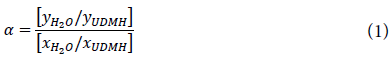Where  and  are weight fractions of water and UDMH in permeate and  and  are weight fractions in feed, respectively [8, 29-31].

MODELING

Fig. 2 represents the schematic diagram of the model domain used in the simulation. A feed solution containing a mixture of 5 wt. % UDMH and 95 wt. % water flows tangentially through the upper side of the membrane system (z=0) and exits at z=L.

The main assumptions to develop the numerical simulation are as follows:

Time-dependent conditions are considered,

Temperature is constant,

No chemical reaction occurs in feed stream,

Feed solution flows only in the z-direction,

Feed flow is laminar in the membrane system,

Thermodynamic equilibrium considered at the interface of feed and membrane,

Small amount of UDMH permeates through the membrane,

Mass transfer resistance of the support layer was assumed to be negligible,

Fouling and concentration polarization effects on the PV of UDMH solution are negligible and

Feed viscosity and density are constant.

Axial and radial diffusions inside the membrane and feed phase are considered in the continuity equations. Moreover, small permeation of UDMH through the membrane is considered in the simulation by applying selectivity equation (Eq. (1)).

Concentration of UDMH in the permeate side () must be determined by trial and error method. In this method, an initial value for  is guessed. Then UDMH concentration in the permeate side is calculated using model equations. This calculated value then is compared with the guessed value.  If the difference between the old and new values is less than a determined error, the guessed UDMH concentration is considered as the correct concentration. Otherwise, another guess must be made for .

Mass transport in the membrane system is described using continuity equation. The following equation presents the differential form of this equation :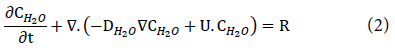Where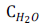denotes water concentration (mol/m3),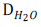denotes water diffusion coefficient (m2/s), U denotes the velocity vector (m/s) and R denotes the reaction term (mol/m3.s). Since no chemical reactions take place in UDMH/water PV, the reaction term is zero. Continuity equation was defined and solved in COMSOL Multiphysics 5.2 by adding a “transport of diluted species” physic to the whole model.

Velocity distribution was obtained by solving Navier-Stokes equation for momentum balance, simultaneously with continuity equation in the feed compartment. This was done by adding a “laminar flow” physic to the whole model in COMSOL Multiphysics 5.2. The following equation describes the momentum conservation equation :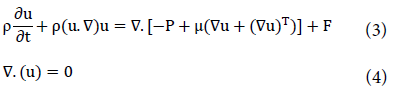Where u denotes z-component of the velocity vector (m/s), ρ denotes feed density (kg/m3), P denotes pressure (Pa),  denotes feed viscosity (Pa.s) and F denotes a body force (N).

Feed phase simulation

By applying mentioned assumptions to Eq. (2), unsteady state form of the continuity equation for water mass transport on the feed side is obtained: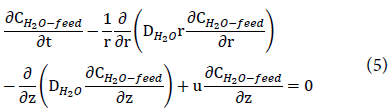Where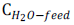is water concentration in feed phase. The simplified form of the momentum transport equations considering above assumptions will be as follows: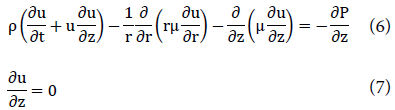Where r and z denote radial and axial coordinates, respectively.

The initial conditions for mass and momentum conservation equations are as follows: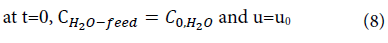Where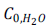is water initial concentration and u0 is initial velocity of feed flow.

The boundary conditions for mass conservation equations in feed phase are as follows: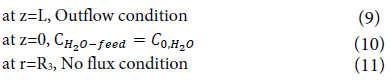Where R3 is the outer radius of the feed section. At the interface of membrane-feed, the equilibrium condition is assumed: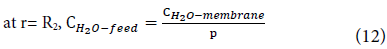In which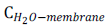is water concentration in membrane section and p is partition coefficient obtained from selectivity equation as follows: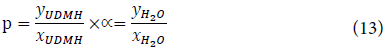As mentioned earlier, permeate concentration of UDMH must be determined using trial and error method and then is placed in the above equation.

The boundary conditions for momentum transfer equations are as follows: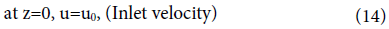At the outlet, the pressure is atmospheric pressure: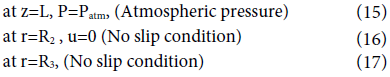Membrane phase simulation

Mass transport of water in the membrane is controlled only by a diffusion mechanism. Therefore, the unsteady state continuity equation for water can be written as: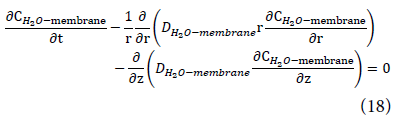Where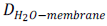is water diffusion coefficient in membrane (m2/s).

Membrane phase boundary conditions are given as: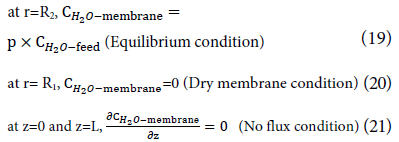At the permeate-membrane interface, water concentration assumed to be zero due to the vacuum applied.

Numerical solution of conservation equations

Set of model equations, including mass and momentum transfer equations in the membrane module along with suitable boundary conditions was solved using COMSOL Multiphysics software version 5.2. Finite element method (FEM) was used by this software to solve conservation equations numerically. The computational time for solving the equations was 336 s. “Extra fine mesh” was used for meshing in this simulation. Complete mesh consisted of 30558 domain elements and 975 boundary elements for solving the set of equations. A number of degrees of freedom were 54564 (plus 1356 internal DOFs). Fig. 3 represents the meshes created by COMSOL Multiphysics 5.2 software. Due to the considerable difference between z and r dimensions, a scaling factor equal to 10 was used in the z-direction. Therefore, the results were reported in dimensionless length.

RESULTS AND DISCUSSION

Previous studies of pervaporation technology showed that PV performance of a dense polymeric membrane relies upon the ability of solvent species to be dissolved in the membrane at its interfaces, and their diffusion into the membrane. When a zeolite membrane is utilized as a filtration tool, the solvent species cannot be dissolved in the membrane phase but they are adsorbed on the zeolite sites of the inorganic materials. Their adsorbed capacities depend on the affinity of the membranes towards the solvents to be removed [33,34].

ZSM-5 performance

The membrane exhibited a high selectivity towards the water in water/UDMH mixtures. The permeate water flux reaches a value as high as 0.67 kg/m2.h for a UDMH concentration of 5 wt. %. The fact that the membrane has a high selectivity towards water clearly indicates that the zeolite layer doesn’t have any through-holes, and the transport is diffusive but not convective. The results also confirm that the ZSM-5 membrane behaves as a hydrophilic membrane, probably due to the presence of polar Al atoms in the zeolite crystal structure.

The ZSM-5 membrane showed a water/UDMH ideal selectivity of 55 at 27oC, indicating its reasonable quality. Even higher selectivity may be expected for higher quality membranes. During PV, water permeates through both zeolite and non-zeolite pores because of its small diameter (the kinetic diameter of water is 0.26 nm). The kinetic diameter of UDMH is larger than that of the zeolite pores, thus, much of the UDMH flux is probably through the non-zeolite pores.

If the zeolite is defect-free, it means that it has no non-zeolite pore and thus, water can pass only through zeolite pores. However, the non-zeolite pores usually exist and are larger than the zeolite pores. Non-zeolite pores have a size distribution and may also affect flux and selectivity. Transport through the non-zeolite pores has contributions from both surface diffusion and Knudsen diffusion, and possibly from viscous flow [35, 36].

The ZSM-5 channel system is shown in Fig. 4. The straight elliptical channels running in the b-direction have the dimensions of 0.53×0.56 nm and the sinusoidal channels running in the a-direction have the dimensions of 0.55×0.51 nm.

Fig. 5 shows XRD patterns of the mullite support and the zeolite membranes. Morphology of the support subjected to crystallization was characterized by SEM (Fig. 6). Fig. 7 shows the morphology of the ZSM-5 membranes (surface and cross-section). As seen, most of the crystals lie disorderly on the surface. The SEM photographs of the membranes (cross-section) show that the mullite surface is completely covered by a zeolite crystal layer, whose thickness is larger than 40 µm. The crystal layer is composed of two layers; the top layer consists of pure ZSM-5crystals and the intermediate one, of ZSM-5crystals grown into the mullite pores.

As seen in Table 1, sample 1 had better selectivity equal to 55 and sample 2 had better flux equal to 2.22 kg/m2.h at 27oC. Furthermore, ZSM-5 membranes prepared using the following gel molar composition: 0.292Na2O: 1.0Al2O3, 100SiO2: 2.0TPABr, 40H2O: 1.0Al2O3, 100SiO2: 1.0Al2O3 showed better results.

Feed phase simulations

Fig. 8 shows the concentration distribution of water in the feed section at different separation times. The UDMH/water solution containing 95 wt. % water flows over the outer surface of the membrane module (z=0). As can be seen from the figure, a concentration boundary layer is formed at the beginning of the separation process. Over an extended period of time, the thickness of this layer increases. At z=0, the water concentration is maximum (95 wt. %). As the feed solution flows in the feed compartment, water moves towards the membrane surface due to the concentration and pressure differences (driving forces). Therefore, the water concentration on the membrane surface is less than its value at the feed inlet (where water concentration is equal to its initial value, ). The water concentration on the membrane surface was calculated from the membrane selectivity (Eq. 12) and its value in the membrane side. Since water concentration in membrane is always less than its value in the feed, the water concentration on membrane-feed boundary (r=R2) is always less than its value in feed bulk. At the feed outlet (z=L) water concentration is minimum, as expected. Over a longer period of separation time, minimum water concentration in concentrate part (feed part) decreases due to the water diffusion through the membrane.

Fig. 9 presents the water concentration in the feed phase versus r-coordinate at different lengths and separation times. Water concentration increases along r direction, as expected. The concentration gradient is great at regions near the membrane-feed interface (r=R2) due to the mass transfer towards the membrane in this region. Over a longer period of time, the total water concentration in feed phase decreases due to the mass transfer towards the membrane. Change in total concentration curves is negligible at 25-75mm membrane lengths. At the regions near the feed outlet the concentration decreases, while its gradient along r direction increases. This can be attributed to lower water concentrations in regions near z=L and areas close to feed-membrane interface compared to feed inlet, as mentioned before, which raises the driving force (concentration gradient). Over an extended period of time, total concentration declines due to mass transfer through the membrane.

Fig. 10 demonstrates the concentration distribution along z coordinate at a constant flow rate (0.5 l/min) and different radii. Results show that the variation of water concentration along the z coordinate is remarkable and cannot be neglected compared to its variation along r coordinate. The figure also indicates that the concentration gradient near the membrane-feed interface (Fig. 10(a)) is slightly greater, while is less and also delayed at larger radii. This behavior can be attributed to water transfer towards the membrane in this region (larger concentration gradients). Concentration variation extends over a larger period of time. Thus, a time-dependent study in PV simulations, which was neglected in previous studies, is necessary [8, 16].

Fig. 11 shows the velocity field in the feed phase of the PV membrane system.  The velocity distribution was obtained using a numerical solution of momentum balance. This was done by adding a “laminar flow” physic to the whole model in COMSOL. As can be seen from the figure, the velocity profile is fully developed after a short distance. Velocity is zero on the membrane-feed interface and outer radius of the feed section boundary (due to no-slip condition).

Fig. 12 illustrates the velocity profile vs. radius in the feed section. As can be seen, the velocity profile is parabolic and becomes fully developed after a short distance (lengths approximately more than 10mm). As seen, entrance effects are considered in this simulation, which is one of the advantages of FEM simulation. Fig. 13 represents velocity distribution vs. dimensionless length. The velocity profile is almost parabolic and reaches its maximum value at the regions close to the feed entrance.

Membrane phase simulation

Fig. 14 shows the concentration distribution of water in membrane phase at different separation times. Water transfer mechanism through the membrane was described only by diffusion.  Since at the membrane-permeate interface the vacuum condition was assumed, the water concentration on this boundary is zero at all separation times. Water concentration is highest on the membrane-feed interface since it is calculated from its value in the feed section, which is greatest in this domain. Over a longer period of time, however, water concentration at the membrane length (z=L) decreases slightly, due to the concentration decrease in feed side (as mentioned in the previous section). These results are very close to what happens in real conditions.

Fig. 15 presents the water concentration in the membrane phase versus r-coordinate at different lengths and times. Water concentration increases with radius, as expected. Concentration value on the membrane-feed boundary (r=R2) is maximum at any time because it is calculated from water concentration in feed side, where its value is maximum. On the membrane-permeate interface, water concentration is zero because of vacuum applied in permeate side (dry membrane condition). The concentration gradient profile is almost the same up to 10 mm membrane lengths. However, in regions near the membrane length (z=100 mm), concentration gradient decreases over an extended period of time. This is because the model considers the loss of concentration in this region and longer periods of time, due to more water transfer towards the membrane at domains close to the feed inlet. As seen, the developed model robustly is capable of predicting this mass loss at z=L and different separation times.

Fig. 16 demonstrates the concentration distribution vs. dimensionless length at a constant flow rate (0.5 l/min) and different membrane’s radii. Results show that the variation of water concentration along the z coordinate at constant radius is considerable and cannot be neglected compared to its variation along r coordinate. Its variation is also greater over an extended period of time, as expected. The total shape of concentration distribution is almost the same at various radii. However, at radii far from the membrane-permeate interface, the concentration increases significantly. This is because water concentration on the membrane-feed interface is calculated from selectivity equation (Eq. 19) and water concentration in feed phase, where its value is highest.

CONCLUSION

ZSM-5 zeolite membranes were firstly used for dehydration of water-UDMH mixtures. The membranes were synthesized on the outer surface of porous mullite tubes by hydrothermal method. The mullite supports were made by extruding kaolin clay. The zeolite membranes showed much higher fluxes and separation factors than commercially available polymeric membranes. The membranes showed good membrane performance for separation of the UDMH-water mixtures. It is expected that even significantly higher fluxes, with similar separation factors, can be achieved at higher temperatures. Performance of PV system was modeled using COMSOL Multiphysics software version 5.2. Modeling was done by solving mass and momentum equations numerically by finite element method (FEM). Good modeling results indicated that FEM is a powerful method for simulating membrane separation systems.

Since the ZSM-5 zeolite membranes can withstand high temperatures and harsh environments (pH>12), dehydration of the water-UDMH mixtures can be performed. It was found that PV using the ZSM-5 zeolite membranes is an effective technique to separate water from the water-UDMH mixtures.

CONFLICT OF INTEREST

The authors declare that there are no conflicts of interest regarding the publication of this manuscript.